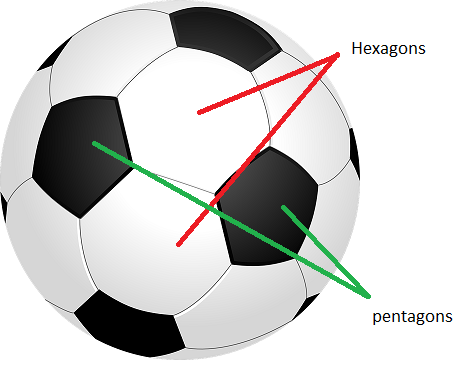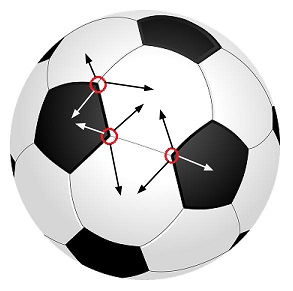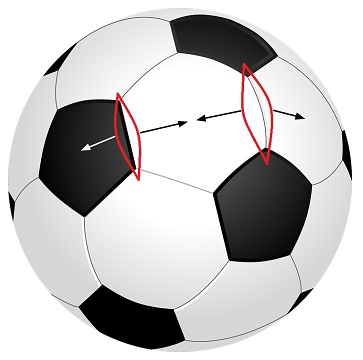# Number of Pentagons and Hexagons on a Football

• Difficulty Level : Hard
• Last Updated : 29 Nov, 2021

Given a standard football, regular hexagons and pentagons are drawn on it as shown in the picture. Find out the number of hexagons and pentagons.## Recommended: Please try your approach on {IDE} first, before moving on to the solution.

We can apply Euler Characteristics to find out number of Hexagons and Pentagons on a standard Football.
According to Euler Characteristics: For every surface S there exists an integersuch that whenever a graph G with V vertices and E edges is embedded in S so that there are F faces(regions divided by the graph), we have:For a sphere(shape of football)= 2.
Hence, the equation becomes V – E + F = 2.

Now, let number of pentagons P and number of hexagons H.

Number of vertices will be:
6 vertices for each of the hexagons, i.e. 6*H.
5 vertices for each of the pentagons, i.e. 5*P.
But we have counted each vertex thrice, once for each adjacent polygon, follow the pictureHence, number of vertices, V = (6*H + 5*P)/3.

Number of edges will be:
6 edges for each of the hexagons, i.e. 6*H.
5 edges for each of the pentagons, i.e. 5*P.
But we have counted each edge twice, once for each adjacent polygon, follow the pictureHence, number of edges, E = (6*H + 5*P)/2.

Number of faces will be:
There are H hexagons and P pentagons, each forming a face. Hence, total number of faces, F = (H + P).

So, we can write:after solving this equation we will get P = 12. So, there are 12 Pentagons.
Now number of Hexagons:
We can see that each pentagon is surrounded by 5 Hexagons. So there should 5*P hexagons, but we have counted each hexagon thrice for each of its 3 adjacent pentagons. Hence, number of hexagons = 5*P/3 = 5*12/3 = 20.

Hence, there are 20 Hexagons and 12 Pentagons in a standard football.

My Personal Notes arrow_drop_up